# AP Statistics : Estimation

## Example Questions

← Previous 1 3

### Example Question #1 : Estimation

What is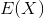, or the expected value offor any distribution?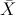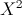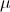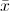Explanation:is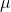, or the mean, of the population. This makes sense sinceliterally means the expected value of. The mean is the expected value ofExplanation:

### Example Question #1 : How To Estimate Margins Of Errors

The next local election is predicted to have a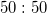split in votes for the top two candidates. How many people should be polled to obtain a margin of error of 3% at the 95% confidence level?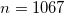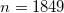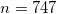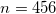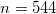Explanation:

If you remember the required formula, this problem is rather simple. Plug in the given numbers and simplify: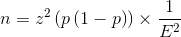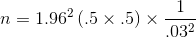If you don't remember the formula, this problem is more challenging. Your best bet in this case is to construct the confidence interval and rearrange to solve for the required sample size.

### Example Question #1 : How To Estimate Margins Of Errors

When dealing with confidence intervals, the margin of error gets smaller when z* gets ________ and n gets _________.

There is no way to affect the margin of error in confidence intervals

smaller . . . smaller

larger . . . smaller

larger . . . larger

smaller . . . larger

smaller . . . larger

Explanation:

For confidence intervals, a small margin of error is preferred, as it indicates that the parameter of interest has been narrowed down to a precise interval. Having a large sample population to work from as well as a small z* (z* gets smaller as the confidence level percent gets lower) can help obtain a small margin of error.

Explanation:

Explanation:

### Example Question #1 : Confidence Intervals And Mean

Suppose you have a normally distributed variable with known variance. How many standard errors do you need to add and subtract from the sample mean so that you obtain 95% confidence intervals?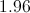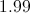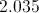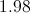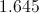Explanation:

To obtain 95% confidence intervals for a normal distribution with known variance, you take the mean and add/subtract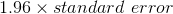. This is because 95% of the values drawn from a normally distributed sampling distribution lie within 1.96 standard errors from the sample mean.

### Example Question #1 : Confidence Intervals And Mean

An automotive engineer wants to estimate the cost of repairing a car that experiences a 25 MPH head-on collision. He crashes 24 cars, and the average repair is $11,000. The standard deviation of the 24-car sample is$2,500.

Provide a 98% confidence interval for the true mean cost of repair.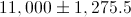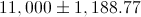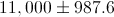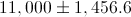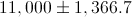Explanation:

Standard deviation for the samle mean: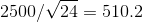Since n < 30, we must use the t-table (not the z-table).

The 98% t-value for n=24 is 2.5.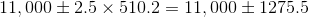### Example Question #1 : How To Find Confidence Intervals For A Mean

300 hundred eggs were randomly chosen from a gravid female salmon and individually weighed. The mean weight was 0.978 g with a standard deviation of 0.042. Find the 95% confidence interval for the mean weight of the salmon eggs (because it is a large n, use the standard normal distribution).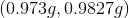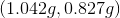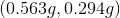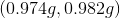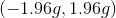Explanation:

Because we have such a large sample size, we are using the standard normal or z-distribution to calculate the confidence interval.

Formula: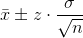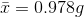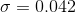We must find the appropriate z-value based on the given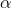for 95% confidence: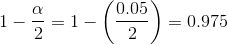Then, find the associated z-score using the z-table for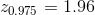Now we fill in the formula with our values from the problem to find the 95% CI.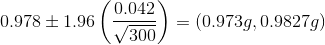### Example Question #2 : Confidence Intervals And Mean

A sample of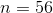observations of 02 consumption by adult western fence lizards gave the following statistics: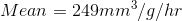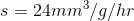Find the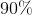confidence limit for the mean 02 consumption by adult western fence lizards.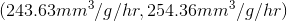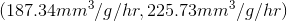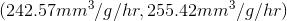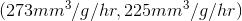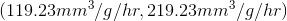Explanation:

Because we are only given the sample standard deviation we will use the t-distribution to calculate the confidence interval.

Appropriate Formula: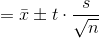Now we must identify our variables: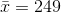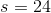We must find the appropriate t-value based on the givent-value at 90% confidence: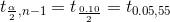Look up t-value for 0.05, 55 , so t-value= ~ 1.6735

90% CI becomes: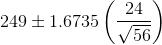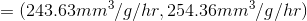← Previous 1 3

### All AP Statistics Resources